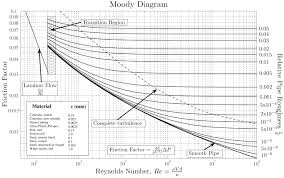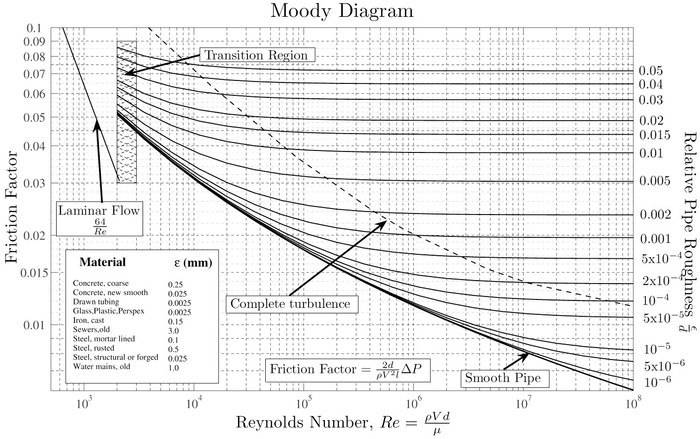# Friction factor at turbulent region

foo9008

## Homework Statement

in my book , the author stated that the friction factor become maximum when the flow become turbulent ...However , according to the Moody Chart , we can know that the friction factor decreases from laminar to turbulent and then constant .... is the statement in the book wrong ?

## Answers and Replies

foo9008Staff Emeritus
Homework Helper

## Homework Statement

in my book , the author stated that the friction factor become maximum when the flow become turbulent ...However , according to the Moody Chart , we can know that the friction factor decreases from laminar to turbulent and then constant .... is the statement in the book wrong ?

It would appear to be so, based on the Moody diagram.

Can you provide a snap shot of the text where the author makes this statement?

•foo9008
Staff Emeritus
Homework Helper
I think the author should have said that the friction factor reaches a minimum when the flow becomes fully turbulent.

•foo9008
Homework Helper
Gold Member
I think the author should have said that the friction factor reaches a minimum when the flow becomes fully turbulent.
Yes, but from the text leading up to it I would say it was not just a slip of the pen. The author really does think it reaches a maximum there.
It does seem paradoxical because, as the author says, the pumping force required is greater at higher velocities. But looking at the v2 term in the Darcy-Weisbach equation, it is clear that some small reduction in friction factor as the velocity increases will not counter that much.

•foo9008
foo9008
Yes, but from the text leading up to it I would say it was not just a slip of the pen. The author really does think it reaches a maximum there.
It does seem paradoxical because, as the author says, the pumping force required is greater at higher velocities. But looking at the v2 term in the Darcy-Weisbach equation, it is clear that some small reduction in friction factor as the velocity increases will not counter that much.
so , it is true that the friction factor will increase when the flow become fully turbulent ? so , the author 's statement that the friction factor will become maximum is wrong ? hwo about the pumping power?

Staff Emeritus
Homework Helper
Yes, but from the text leading up to it I would say it was not just a slip of the pen. The author really does think it reaches a maximum there.
It does seem paradoxical because, as the author says, the pumping force required is greater at higher velocities. But looking at the v2 term in the Darcy-Weisbach equation, it is clear that some small reduction in friction factor as the velocity increases will not counter that much.
While I agree that the total friction varies as flow velocity squared, the author doesn't say that the total friction is a maximum for fully turbulent flow; instead he says the friction factor is a maximum. That distinction is the one thing which operates in the author's favor.

The Moody diagram, which plots friction factor versus Reynold's No., and by extension flow velocity, shows that the friction factor assumes a constant value which is independent of the Reynold's No. of the flow and hence the flow velocity for fully turbulent flow. That's what all those horizontal lines indicate on this diagram:For a given value of relative pipe roughness, the friction factor takes on a relative maximum value somewhere in the transition zone between fully laminar and fully turbulent flow. Granted, the Reynold's No. at which the different types of flow appear seems to be somewhat arbitrary, having a minimum friction factor in the turbulent zone is quite obvious from the Moody diagram.

Homework Helper
Gold Member
so , it is true that the friction factor will increase when the flow become fully turbulent ?
No.

, the author 's statement that the friction factor will become maximum is wrong
Yes.

Staff Emeritus
Homework Helper
so , it is true that the friction factor will increase when the flow become fully turbulent ?
No. Read my Post #8 above and look at the Moody diagram.

so , the author 's statement that the friction factor will become maximum is wrong ?
Yes, obviously.
hwo about the pumping power?
The pumping power is still directly proportional to the pressure drop and the flow rate.

Homework Helper
Gold Member
the total friction varies as flow velocity squared, the author doesn't say that the total friction is a maximum for fully turbulent flow; instead he says the friction factor is a maximum. That distinction is the one thing which operates in the author's favor.
No, it operates against the author. The total friction increases as the velocity increases, but the friction factor decreases.

foo9008
so
While I agree that the total friction varies as flow velocity squared, the author doesn't say that the total friction is a maximum for fully turbulent flow; instead he says the friction factor is a maximum. That distinction is the one thing which operates in the author's favor.

The Moody diagram, which plots friction factor versus Reynold's No., and by extension flow velocity, shows that the friction factor assumes a constant value which is independent of the Reynold's No. of the flow and hence the flow velocity for fully turbulent flow. That's what all those horizontal lines indicate on this diagram:For a given value of relative pipe roughness, the friction factor takes on a relative maximum value somewhere in the transition zone between fully laminar and fully turbulent flow. Granted, the Reynold's No. at which the different types of flow appear seems to be somewhat arbitrary, having a minimum friction factor in the turbulent zone is quite obvious from the Moody diagram.
While I agree that the total friction varies as flow velocity squared, the author doesn't say that the total friction is a maximum for fully turbulent flow; instead he says the friction factor is a maximum. That distinction is the one thing which operates in the author's favor.

The Moody diagram, which plots friction factor versus Reynold's No., and by extension flow velocity, shows that the friction factor assumes a constant value which is independent of the Reynold's No. of the flow and hence the flow velocity for fully turbulent flow. That's what all those horizontal lines indicate on this diagram:For a given value of relative pipe roughness, the friction factor takes on a relative maximum value somewhere in the transition zone between fully laminar and fully turbulent flow. Granted, the Reynold's No. at which the different types of flow appear seems to be somewhat arbitrary, having a minimum friction factor in the turbulent zone is quite obvious from the Moody diagram.
so , correct statement should be , the total friction become maximum ,while the frictional factor become minimum ?

Homework Helper
Gold Member
so

so , correct statement should be , the total friction become maximum ,while the frictional factor become minimum ?
Yes.

foo9008
Yes.
so , beside the friction factor that cause the friction force , there are other fricition forces acting on it ?

Staff Emeritus
Homework Helper
so , beside the friction factor that cause the friction force , there are other fricition forces acting on it ?
You'll have to be more specific.

foo9008
You'll have to be more specific.
what do you mean ?

Staff Emeritus
Homework Helper
what do you mean ?
The pressure drop formula is:

PD = f (L/D) v2 / 2g

Except for g, everything else can be a variable.

so , beside the friction factor that cause the friction force , there are other fricition forces acting on it ?

Other friction forces acting on what exactly? That's what is not clear.

foo9008
The pressure drop formula is:

PD = f (L/D) v2 / 2g

Except for g, everything else can be a variable.

Other friction forces acting on what exactly? That's what is not clear.
on fluid and pipe

Staff Emeritus
Homework Helper
on fluid and pipe
Doesn't seem to be for simple incompressible flows.

That's not to say that you could wind up with some wild, compressible flow situation with a lot of externally applied forces, temperature changes, phase changes, etc., but why go looking for trouble?

•